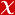## Measurement of the top quark pair production cross section in dilepton final states containing one tau lepton in pp collisions at root s=13 TeV

JOURNAL OF HIGH ENERGY PHYSICS, no.2, 2020 (Journal Indexed in SCI)• Publication Type: Article / Article
• Volume: Issue: 2
• Publication Date: 2020
• Doi Number: 10.1007/jhep02(2020)191
• Title of Journal : JOURNAL OF HIGH ENERGY PHYSICS

#### Abstract

The cross section of top quark pair production is measured in the t (t) over bar -> (lv(l))(tau(h)v(tau))b (b) over bar final state, where tau(h) refers to the hadronic decays of the tau lepton, and l is either an electron or a muon. The data sample corresponds to an integrated luminosity of 35.9 fb(-1) collected in proton-proton collisions at root s = 13TeV with the CMS detector. The measured cross section is sigma(t (t) over bar) = 781 +/- 7 (stat) +/- 62 (syst) +/- 20 (lumi) pb, and the ratio of the partial width Gamma(t -> tau v(tau)b) to the total decay width of the top quark is measured to be 0.1050 +/- 0.0009 (stat) +/- 0.0071 (syst). This is the first measurement of the t (t) over bar production cross section in proton-proton collisions at root s = 13TeV that explicitly includes t leptons. The ratio of the cross sections in the l tau(h) and ll final states yields a value R-l tau h/ll = 0.973 +/- 0.009 (stat) +/- 0.066 (syst), consistent with lepton universality.